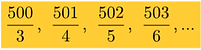Fractions

Let's Reduce!

Which of the following choices is correct?

a.) No fractions can be reduced.

b.) One fraction can be reduced.

c.) Two fractions can be reduced.

d.) More than three fractions can be reduced.Polygons & Fractions

Among all n-sided polygons with the same perimeter, the regular polygon has the largest area.Use the rule above to determine which fraction is larger:

Fractions to Integers

How many fractions in the following sequence simplify to integers?

a.) One fraction

b.) Two fractions

c.) Three fractions

d.) More than three fractions2020 in Fractions

Find three different integers that satisfy the following equation.Is more than one solution possible?